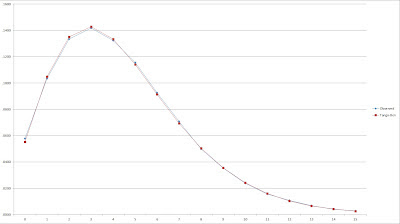## Monday, June 25, 2012

### On Run Distributions, pt. 4: Tango Distribution

At this point in the series, I need to take a detour from discussing run per game distributions and get a little more fundamental--runs per inning distributions. Thankfully, this will not involve any painful attempts to develop a model, as a good one already exists: the Tango Distribution.

In order to implement the zero-modified negative binomial model I’ve discussed, I need a way to estimate the probability of zero runs being scored in a game. While converting a runs per inning distribution to a runs per game distribution involves a mess of combination math, estimating the probability of a shutout from an inning distribution is much simpler. Theoretically, the probability of a shutout should be the probability of failing to score in a single inning to the ninth power (that is, the probability of failing to score in nine consecutive innings).

Of course, this requires making some assumptions, most notably that there are nine innings per game (in fact, if both teams fail to score, then in at least 50% of such games a team will fail to score in the first nine innings and still not get whitewashed for the game) and that the run distribution in each inning is independent.

The Tango Distribution uses Runs/Inning (RI) as one parameter, and a control value c as the other. c is set equal to .767 if looking at one team independently and .852 for teams in a head-to-head game. Then:

a = c*RI^2
f(0) = RI/(RI + a)
d = 1 - c*f(0)
f(1) = (1 - f(0))*(1 - d)
f(k) = f(k - 1)*d for k >= 2

I’ll assume that RI = (R/G)/9, so if we have a team that averages 4.4558 R/G, their RI = .4951.

a = .767*.4951^2 = .188
f(0) = .4951/(.4951 + .188) = .7248
probability(0 runs in game) = p(0) = f(0)^9 = .7248^9 = .0552

Our empirical probability of a shutout for teams averaging 4.4558 R/G was .0578.

We will use the Tango Distribution to estimate the parameter z for the Enby distribution. I’ll restate the formula here, omitting the superfluous formulas for the probabilities of scoring in the inning:

z = (RI/(RI + c*RI^2))^9 where default is c = .767

As I mentioned earlier, Ben Vollmayr-Lee demonstrated how to use the Tango Distribution to estimate the per game run distribution, assuming that runs are independent across innings. His explanation is available here (zip link).

This provides us an alternative means of estimating the runs per game distribution. It’s really not important to me at this whether the converted Tango Distribution or the Enby distribution does better, as the latter is easier to implement and I’ve surely not been able to optimize it. In any event, the Tango Distribution is a very valuable tool to have as well, certainly for estimating the runs per inning distribution but also for the runs per game distribution.

I have a spreadsheet which implements Vollmayr-Lee’s approach, and used it to generate estimated runs per game distributions for the three samples I’ve referenced throughout the series. I’ll give you the graphs here as I did for the Enby distribution. First, for all teams 1981-1996:On the eyeball test, it certainly does seem as if the Tango-Ben method is a better fit than the Enby distribution, especially when we consider that the latter has a number of artificial advantages in this test. Here are the 25 lowest scoring teams:This doesn’t look as good, but remember the sample size caveat and it still looks a little better than the Enby distribution. For the 25 highest scoring teams:Pretty good, again appearing to be a better fit than Enby. Again, I want to stress that I don’t think Enby is any kind of silver bullet; its potential value is due to its being a distribution that returns a direct result in terms of runs per game and is a well-known distribution.